0%

# Binary Tree based Recursion & Divide Conquer 二叉树递归与分治

### 226. Invert Binary Tree (Easy)

Invert a binary tree.

Trivia:
This problem was inspired by this original tweet by Max Howell:
Google: 90% of our engineers use the software you wrote (Homebrew), but you can’t invert a binary tree on a whiteboard so f*** off.

### 100. Same Tree (Easy)

Given two binary trees, write a function to check if they are the same or not.
Two binary trees are considered the same if they are structurally identical and the nodes have the same value.

### 101. Symmetric Tree (Easy)

Given a binary tree, check whether it is a mirror of itself (ie, symmetric around its center).

Note:
Bonus points if you could solve it both recursively and iteratively.

8刷：高频

### 104. Maximum Depth of Binary Tree (Easy)

Given a binary tree, find its maximum depth.
The maximum depth is the number of nodes along the longest path from the root node down to the farthest leaf node.
Note: A leaf is a node with no children.

8刷：高频

### 111. Minimum Depth of Binary Tree (Easy)

again! 递归，遍历已掌握
Given a binary tree, find its minimum depth.
The minimum depth is the number of nodes along the shortest path from the root node down to the nearest leaf node.
Note: A leaf is a node with no children.
Example:

### 144. Binary Tree Preorder Traversal (Medium)

again!
Given a binary tree, return the preorder traversal of its nodes’ values.

Follow up: Recursive solution is trivial, could you do it iteratively?

### 94. Binary Tree Inorder Traversal (Medium)

Given a binary tree, return the inorder traversal of its nodes’ values.
Example:

Follow up: Recursive solution is trivial, could you do it iteratively?

### 230. Kth Smallest Element in a BST (Medium)

Given a binary search tree, write a function kthSmallest to find the kth smallest element in it.
Note:
You may assume k is always valid, 1 ≤ k ≤ BST’s total elements.

What if the BST is modified (insert/delete operations) often and you need to find the kth smallest frequently? How would you optimize the kthSmallest routine?

10刷：面经，Triplebyte, 维萨
Follow up: 二叉树经常被修改 如何优化 kthSmallest 这个操作? leetcode官方答案提出做一个类似LRU双链表的数据结构实现O(h + k)插删和查询

### 129. Sum Root to Leaf Numbers (Medium)

again!
Given a binary tree containing digits from 0-9 only, each root-to-leaf path could represent a number.
An example is the root-to-leaf path 1->2->3 which represents the number 123.
Find the total sum of all root-to-leaf numbers.
Note: A leaf is a node with no children.

### 116. Populating Next Right Pointers in Each Node (Medium)

You are given a perfect binary tree where all leaves are on the same level, and every parent has two children. The binary tree has the following definition:

Populate each next pointer to point to its next right node. If there is no next right node, the next pointer should be set to NULL.
Initially, all next pointers are set to NULL.
Example: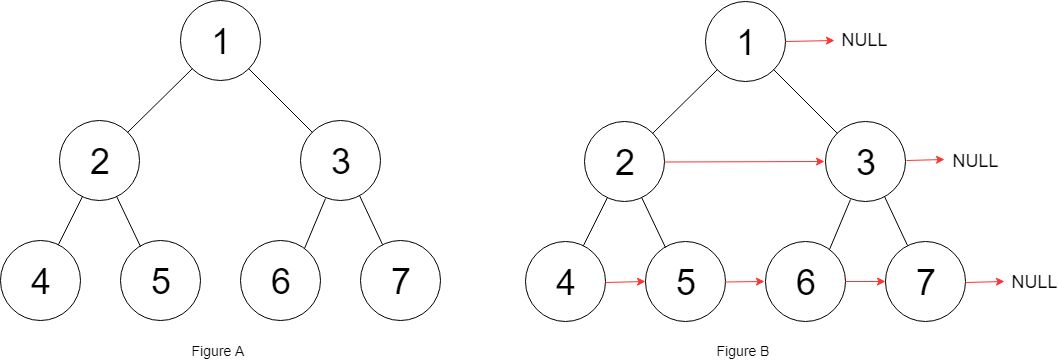### 117. Populating Next Right Pointers in Each Node II (Medium)

again!
Given a binary tree

Populate each next pointer to point to its next right node. If there is no next right node, the next pointer should be set to NULL.
Initially, all next pointers are set to NULL.
Example: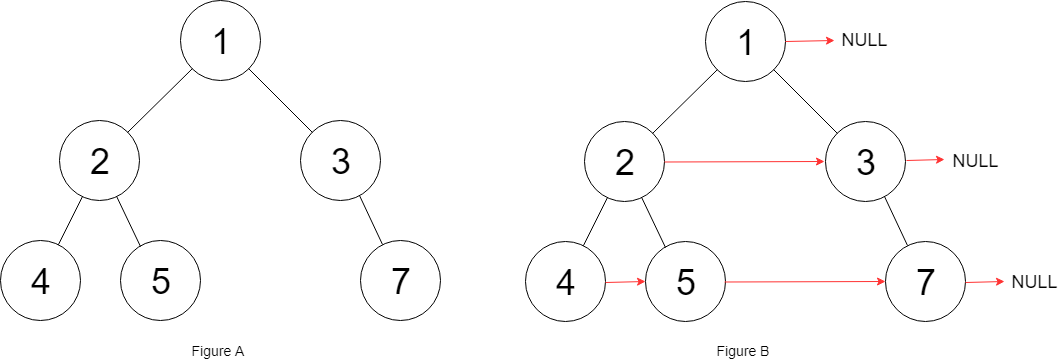Note:
You may only use constant extra space.
Recursive approach is fine, implicit stack space does not count as extra space for this problem.

### 110. Balanced Binary Tree (Easy)

again!
Given a binary tree, determine if it is height-balanced.
For this problem, a height-balanced binary tree is defined as:
a binary tree in which the depth of the two subtrees of every node never differ by more than 1.

### 108. Convert Sorted Array to Binary Search Tree (Easy)

Given an array where elements are sorted in ascending order, convert it to a height balanced BST.
For this problem, a height-balanced binary tree is defined as a binary tree in which the depth of the two subtrees of every node never differ by more than 1.

Example:
Given the sorted array: [-10,-3,0,5,9],
One possible answer is: [0,-3,9,-10,null,5], which represents the following height balanced BST:

### 109. Convert Sorted List to Binary Search Tree (Medium)

again!
Given a singly linked list where elements are sorted in ascending order, convert it to a height balanced BST.
For this problem, a height-balanced binary tree is defined as a binary tree in which the depth of the two subtrees of every node never differ by more than 1.

Example:
Given the sorted linked list: [-10,-3,0,5,9],
One possible answer is: [0,-3,9,-10,null,5], which represents the following height balanced BST:

### 112. Path Sum (Easy)

Given a binary tree and a sum, determine if the tree has a root-to-leaf path such that adding up all the values along the path equals the given sum.
Note: A leaf is a node with no children.

Example:
Given the below binary tree and sum = 22,

### 199. Binary Tree Right Side View (Medium)

Given a binary tree, imagine yourself standing on the right side of it, return the values of the nodes you can see ordered from top to bottom.

6刷：面经, 维萨

### 235. Lowest Common Ancestor of a Binary Search Tree (Easy)

Given a binary search tree (BST), find the lowest common ancestor (LCA) of two given nodes in the BST.
According to the definition of LCA on Wikipedia: “The lowest common ancestor is defined between two nodes p and q as the lowest node in T that has both p and q as descendants (where we allow a node to be a descendant of itself).”
Given binary search tree: root = [6,2,8,0,4,7,9,null,null,3,5]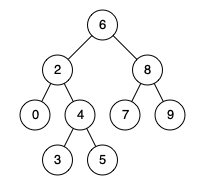Example 1:
Input: root = [6,2,8,0,4,7,9,null,null,3,5], p = 2, q = 8
Output: 6
Explanation: The LCA of nodes 2 and 8 is 6.

Example 2:
Input: root = [6,2,8,0,4,7,9,null,null,3,5], p = 2, q = 4
Output: 2
Explanation: The LCA of nodes 2 and 4 is 2, since a node can be a descendant of itself according to the LCA definition.

Note:
All of the nodes’ values will be unique.
p and q are different and both values will exist in the BST.

### 543. Diameter of Binary Tree (Easy)

Given a binary tree, you need to compute the length of the diameter of the tree. The diameter of a binary tree is the length of the longest path between any two nodes in a tree. This path may or may not pass through the root.

Note: The length of path between two nodes is represented by the number of edges between them.

6刷： O(n), 重点是理解题的本质是求左右子树的maxDepth, 每一步都算一下当前l+r是否大于ans

### 563. Binary Tree Tilt (Easy)

Given a binary tree, return the tilt of the whole tree.
The tilt of a tree node is defined as the absolute difference between the sum of all left subtree node values and the sum of all right subtree node values. Null node has tilt 0.
The tilt of the whole tree is defined as the sum of all nodes’ tilt.

Example:

Explanation:
Tilt of node 2 : 0
Tilt of node 3 : 0
Tilt of node 1 : |2-3| = 1
Tilt of binary tree : 0 + 0 + 1 = 1
Note:
The sum of node values in any subtree won’t exceed the range of 32-bit integer.
All the tilt values won’t exceed the range of 32-bit integer.

### 669. Trim a Binary Search Tree (Easy)

Given a binary search tree and the lowest and highest boundaries as L and R, trim the tree so that all its elements lies in [L, R] (R >= L). You might need to change the root of the tree, so the result should return the new root of the trimmed binary search tree.

10刷：每层三种情况：1：小于最小，则返回右子树传上来的root；2：大于最大，则返回左子树传上来的root；3.大小之间，将root返回上层

### 687. Longest Univalue Path (Medium)

Given a binary tree, find the length of the longest path where each node in the path has the same value. This path may or may not pass through the root.
The length of path between two nodes is represented by the number of edges between them.

Note: The given binary tree has not more than 10000 nodes. The height of the tree is not more than 1000.

16刷：写法1：l, r左右各自递归后lp = rp = 0; if …: lp = l + 1…ans = max(ans, lp + rp) return max(lp, rp)。写法2：f(root, pre) 当前层无法判断是否左右子节点都相等，但是因为如果有一边子节点不相等会将l或r返回为0，那么ans = max(ans, l + r)即可获得想要的答案

### 114. Flatten Binary Tree to Linked List (Medium)

Given a binary tree, flatten it to a linked list in-place.

### 236. Lowest Common Ancestor of a Binary Tree (Medium)

Given a binary tree, find the lowest common ancestor (LCA) of two given nodes in the tree.
According to the definition of LCA on Wikipedia: “The lowest common ancestor is defined between two nodes p and q as the lowest node in T that has both p and q as descendants (where we allow a node to be a descendant of itself).”
Given the following binary tree: root = [3,5,1,6,2,0,8,null,null,7,4]

Example 1:
Input: root = [3,5,1,6,2,0,8,null,null,7,4], p = 5, q = 1
Output: 3
Explanation: The LCA of of nodes 5 and 1 is 3.

Example 2:
Input: root = [3,5,1,6,2,0,8,null,null,7,4], p = 5, q = 4
Output: 5
Explanation: The LCA of nodes 5 and 4 is 5, since a node can be a descendant of itself
according to the LCA definition.

Note:
All of the nodes’ values will be unique.
p and q are different and both values will exist in the binary tree.

13刷：递归：注意最后返回root需要if l and r这个条件。遍历：遍历，建立parent关系，p存入ancestors，遍历p的parent入ancestors，遍历q和q的parent，在ancestors中即返回。TODO 不需要parent关系的算法

### 285. Inorder Successor in BST (Medium) 带锁

LinC 448. Inorder Successor in BST (Medium)
Given a binary search tree and a node in it, find the in-order successor of that node in the BST.
If the given node has no in-order successor in the tree, return null.
It’s guaranteed p is one node in the given tree. (You can directly compare the memory address to find p)

Challenge
O(h), where h is the height of the BST.

O(h)

O(n):

O(n)遍历：

O(n)递归2：

13刷：递归O(n)解法：注意：处理p == inPre需要在递归调用左子树之后，否则pre为空；找到ans以后如果想return需要将inPre = None，否则会锁定inPre，导致上层的 inPre == p，而将上层的root赋值到ans中。
O(h)解法：找p的successor，那么如果root比p小p在右子树，反之p在左子树，去左子树之前要记一下suc节点，以防找到的root.val == p.val节点无右节点（即需要返回上层的suc）。如有右节点，则返回右节点中最小的（往右走一个然后返回最左节点）

### 98. Validate Binary Search Tree (Medium)

Given a binary tree, determine if it is a valid binary search tree (BST).
Assume a BST is defined as follows:
The left subtree of a node contains only nodes with keys less than the node’s key.
The right subtree of a node contains only nodes with keys greater than the node’s key.
Both the left and right subtrees must also be binary search trees.

inorder 中序遍历无额外数组：

inorder 中序递归无额外数组：

inorder 中序递归额外数组：

8刷：高频，五种解法：1.利用BST性质的递归，2，3.中序遍历递归（有/无额外数组），4，5.中序遍历遍历（有/无额外数组）

### 572. Subtree of Another Tree (Easy)

Given two non-empty binary trees s and t, check whether tree t has exactly the same structure and node values with a subtree of s. A subtree of s is a tree consists of a node in s and all of this node’s descendants. The tree s could also be considered as a subtree of itself.

8刷：高频

### 606. Construct String from Binary Tree (Easy)

You need to construct a string consists of parenthesis and integers from a binary tree with the preorder traversing way.
The null node needs to be represented by empty parenthesis pair “()”. And you need to omit all the empty parenthesis pairs that don’t affect the one-to-one mapping relationship between the string and the original binary tree.

11刷

### 297. Serialize and Deserialize Binary Tree (Hard)

Serialization is the process of converting a data structure or object into a sequence of bits so that it can be stored in a file or memory buffer, or transmitted across a network connection link to be reconstructed later in the same or another computer environment.
Design an algorithm to serialize and deserialize a binary tree. There is no restriction on how your serialization/deserialization algorithm should work. You just need to ensure that a binary tree can be serialized to a string and this string can be deserialized to the original tree structure.

Clarification: The above format is the same as how LeetCode serializes a binary tree. You do not necessarily need to follow this format, so please be creative and come up with different approaches yourself.
Note: Do not use class member/global/static variables to store states. Your serialize and deserialize algorithms should be stateless.

dfs递归：

bfs：

dfs遍历：

17刷

### 536. Construct Binary Tree from String (Medium) 带锁

LinC 880. Construct Binary Tree from String (Medium)
You need to construct a binary tree from a string consisting of parenthesis and integers.
The whole input represents a binary tree. It contains an integer followed by zero, one or two pairs of parenthesis. The integer represents the root’s value and a pair of parenthesis contains a child binary tree with the same structure.
You always start to construct the left child node of the parent first if it exists.

Notice
There will only be ‘(‘, ‘)’, ‘-‘ and ‘0’ ~ ‘9’ in the input string.
An empty tree is represented by “” instead of “()”.

### 105. Construct Binary Tree from Preorder and Inorder Traversal (Medium)

Given preorder and inorder traversal of a tree, construct the binary tree.
Note:
You may assume that duplicates do not exist in the tree.

For example, given
preorder = [3,9,20,15,7]
inorder = [9,3,15,20,7]
Return the following binary tree:

7刷：高频，O(n)时间和空间

### 106. Construct Binary Tree from Inorder and Postorder Traversal (Medium)

Given inorder and postorder traversal of a tree, construct the binary tree.
Note:
You may assume that duplicates do not exist in the tree.

For example, given
inorder = [9,3,15,20,7]
postorder = [9,15,7,20,3]
Return the following binary tree:

O(n^2) time and space:

O(n) time and space:

### 889. Construct Binary Tree from Preorder and Postorder Traversal (Medium)

Return any binary tree that matches the given preorder and postorder traversals.
Values in the traversals pre and post are distinct positive integers.

Example 1:
Input: pre = [1,2,4,5,3,6,7], post = [4,5,2,6,7,3,1]
Output: [1,2,3,4,5,6,7]

Note:
1 <= pre.length == post.length <= 30
pre[] and post[] are both permutations of 1, 2, …, pre.length.
It is guaranteed an answer exists. If there exists multiple answers, you can return any of them.

O(n)时间和空间解：

### 865. Smallest Subtree with all the Deepest Nodes (Medium)

Given a binary tree rooted at root, the depth of each node is the shortest distance to the root.
A node is deepest if it has the largest depth possible among any node in the entire tree.
The subtree of a node is that node, plus the set of all descendants of that node.
Return the node with the largest depth such that it contains all the deepest nodes in its subtree.

Example 1:
Input: [3,5,1,6,2,0,8,null,null,7,4]
Output: [2,7,4]
Explanation: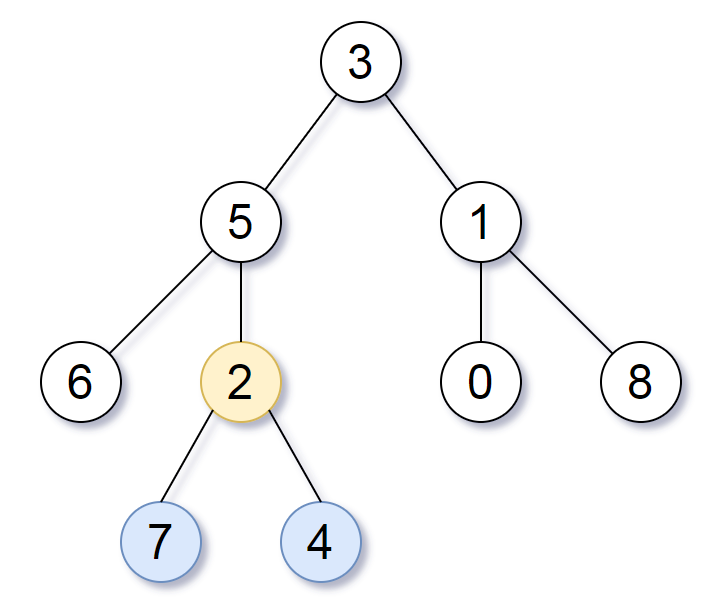We return the node with value 2, colored in yellow in the diagram.
The nodes colored in blue are the deepest nodes of the tree.
The input “[3, 5, 1, 6, 2, 0, 8, null, null, 7, 4]” is a serialization of the given tree.
The output “[2, 7, 4]” is a serialization of the subtree rooted at the node with value 2.
Both the input and output have TreeNode type.

Note:
The number of nodes in the tree will be between 1 and 500.
The values of each node are unique.

### 426. Convert Binary Search Tree to Sorted Doubly Linked List (Medium) 带锁

Linc 1534. Convert Binary Search Tree to Sorted Doubly Linked List

Convert a BST to a sorted circular doubly-linked list in-place. Think of the left and right pointers as synonymous to the previous and next pointers in a doubly-linked list.
Let’s take the following BST as an example, it may help you understand the problem better: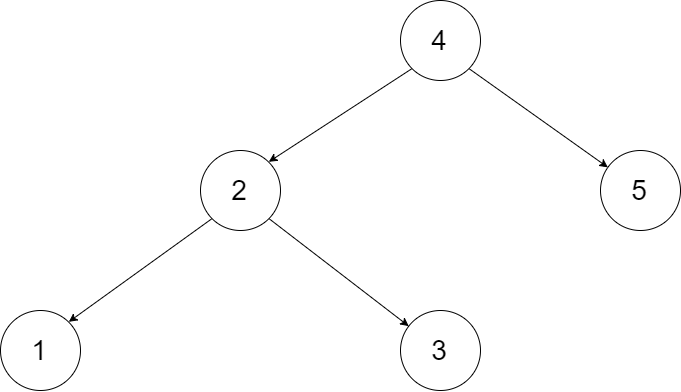We want to transform this BST into a circular doubly linked list. Each node in a doubly linked list has a predecessor and successor. For a circular doubly linked list, the predecessor of the first element is the last element, and the successor of the last element is the first element.
The figure below shows the circular doubly linked list for the BST above. The “head” symbol means the node it points to is the smallest element of the linked list.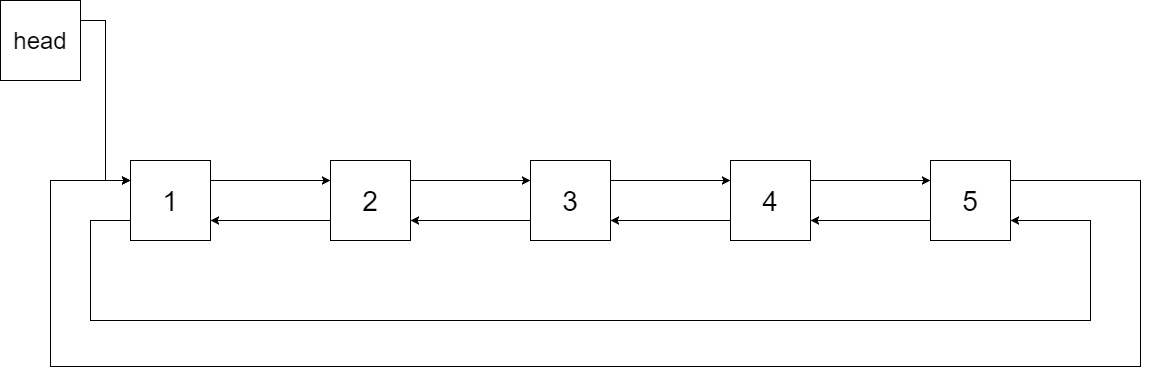Specifically, we want to do the transformation in place. After the transformation, the left pointer of the tree node should point to its predecessor, and the right pointer should point to its successor. We should return the pointer to the first element of the linked list.
The figure below shows the transformed BST. The solid line indicates the successor relationship, while the dashed line means the predecessor relationship.### 298. Binary Tree Longest Consecutive Sequence (Medium) 带锁

LinC 595. Binary Tree Longest Consecutive Sequence
Given a binary tree, find the length of the longest consecutive sequence path.
The path refers to any sequence of nodes from some starting node to any node in the tree along the parent-child connections. The longest consecutive path need to be from parent to child (cannot be the reverse).

### 897. Increasing Order Search Tree (Easy)

Given a binary search tree, rearrange the tree in in-order so that the leftmost node in the tree is now the root of the tree, and every node has no left child and only 1 right child.

Note:
The number of nodes in the given tree will be between 1 and 100.
Each node will have a unique integer value from 0 to 1000.

## Tree based BFS 基于树的 BFS

### 102. Binary Tree Level Order Traversal (Medium)

Given a binary tree, return the level order traversal of its nodes’ values. (ie, from left to right, level by level).

### 107. Binary Tree Level Order Traversal II (Easy)

Given a binary tree, return the bottom-up level order traversal of its nodes’ values. (ie, from left to right, level by level from leaf to root).

### 103. Binary Tree Zigzag Level Order Traversal (Medium)

LinC 71. Binary Tree Zigzag Order Traversal
Given a binary tree, return the zigzag level order traversal of its nodes’ values. (ie, from left to right, then right to left for the next level and alternate between).

DFS:

BFS:

3刷：高频

### 958. Check Completeness of a Binary Tree (Medium)

Given a binary tree, determine if it is a complete binary tree.
Definition of a complete binary tree from Wikipedia:
In a complete binary tree every level, except possibly the last, is completely filled, and all nodes in the last level are as far left as possible. It can have between 1 and 2h nodes inclusive at the last level h.

Example 1: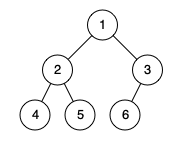Input: [1,2,3,4,5,6]
Output: true
Explanation: Every level before the last is full (ie. levels with node-values {1} and {2, 3}), and all nodes in the last level ({4, 5, 6}) are as far left as possible.

Example 2: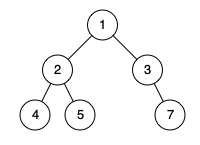Input: [1,2,3,4,5,null,7]
Output: false
Explanation: The node with value 7 isn’t as far left as possible.

Note:
The tree will have between 1 and 100 nodes.

# Binary Search & LogN Algorithm

### 704. Binary Search (Easy)

lintcode’s version

3刷: 高频

### 34. Find First and Last Position of Element in Sorted Array (Medium)

3刷：高频，二分法找 Target, 两次二分法，一次找左边界，一次找右边界

### 852. Peak Index in a Mountain Array

LinC 585. Maximum Number in Mountain Sequence (Medium)
Given a mountain sequence of n integers which increase firstly and then decrease, find the mountain top.
Example
Given nums = [1, 2, 4, 8, 6, 3] return 8
Given nums = [10, 9, 8, 7], return 10

### 162. Find Peak Element (Medium)

A peak element is an element that is greater than its neighbors.
Given an input array nums, where nums[i] ≠ nums[i+1], find a peak element and return its index.
The array may contain multiple peaks, in that case return the index to any one of the peaks is fine.
You may imagine that nums[-1] = nums[n] = -∞.

Example 1:
Input: nums = [1,2,3,1]
Output: 2
Explanation: 3 is a peak element and your function should return the index number 2.

Example 2:
Input: nums = [1,2,1,3,5,6,4]
Output: 1 or 5
Explanation: Your function can return either index number 1 where the peak element is 2,
or index number 5 where the peak element is 6.
Note:
Your solution should be in logarithmic complexity.

2刷：面经，Quora。关键要知道切中点，如果是///向上，则顶点在右，如果/^\则找到顶点，否则顶点在左

3刷：高频

### 153. Find Minimum in Rotated Sorted Array (Medium)

Follow up: 如果有重复的数? 无法保证在 Log(N) 的时间复杂度内解决 例子:[1,1,1,1,1….,1] 里藏着一个 0.最坏情况下需要把每个位置上的1都看一遍，才能找到最后一个有0 的位置. 考点:能想到这个最坏情况的例子

### 33. Search in Rotated Sorted Array (Medium)

3刷： 高频：…while l + 1 < r…

### 658. Find K Closest Elements (Medium)

LinC 460. Find K Closest Elements (Medium)

4刷：二分查找 target，将 l, r 指针放到离x最近的位置…if arr[mid] **<** x: l = **mid** else: r = **mid**…；然后开始往结果里填充k个数

2刷：高频

use bisect:

4刷：高频

# Two pointers

### 283. Move Zeroes (Easy)

Given an array nums, write a function to move all 0’s to the end of it while maintaining the relative order of the non-zero elements.

Example:
Input: [0,1,0,3,12]
Output: [1,3,12,0,0]

Note:
You must do this in-place without making a copy of the array.
Minimize the total number of operations.

6刷：高频：l，r从0，0开始，l，r永远前进，省去很多判断的麻烦

3刷，高频

### 1. Two Sum (Easy)

Given an array of integers, return indices of the two numbers such that they add up to a specific target.
You may assume that each input would have exactly one solution, and you may not use the same element twice.

Example:
Given nums = [2, 7, 11, 15], target = 9,
Because nums + nums = 2 + 7 = 9,
return [0, 1].

### 15. 3Sum (Medium)

Given an array nums of n integers, are there elements a, b, c in nums such that a + b + c = 0? Find all unique triplets in the array which gives the sum of zero.
Note:
The solution set must not contain duplicate triplets.

O(n^2)：

7刷：面经：维萨

### LinC 31. Partition Array (Medium)

Description
Given an array nums of integers and an int k, partition the array (i.e move the elements in “nums”) such that:
All elements < k are moved to the left
All elements >= k are moved to the right
Return the partitioning index, i.e the first index i nums[i] >= k.
You should do really partition in array nums instead of just counting the numbers of integers smaller than k.
If all elements in nums are smaller than k, then return nums.length

Example
If nums = [3,2,2,1] and k=2, a valid answer is 1.

Challenge
Can you partition the array in-place and in O(n)?

### 215. Kth Largest Element in an Array (Medium)

Find the kth largest element in an unsorted array. Note that it is the kth largest element in the sorted order, not the kth distinct element.
Example 1:
Input: [3,2,1,5,6,4] and k = 2
Output: 5

Example 2:
Input: [3,2,3,1,2,4,5,5,6] and k = 4
Output: 4
Note:
You may assume k is always valid, 1 ≤ k ≤ array’s length.

4刷，由于完全抛弃另一侧，时间复杂度平均由 quick sort 的 O(nlogn) 降为 O(n) 因为输入变小了， quicksort 的输入一直是 n, 最差情况 O(n^2)

### 75. Sort Colors (Medium)

Given an array with n objects colored red, white or blue, sort them in-place so that objects of the same color are adjacent, with the colors in the order red, white and blue.
Here, we will use the integers 0, 1, and 2 to represent the color red, white, and blue respectively.

Note: You are not suppose to use the library’s sort function for this problem.

Example:
Input: [2,0,2,1,1,0]
Output: [0,0,1,1,2,2]

A rather straight forward solution is a two-pass algorithm using counting sort.
First, iterate the array counting number of 0’s, 1’s, and 2’s, then overwrite array with total number of 0’s, then 1’s and followed by 2’s.
Could you come up with a one-pass algorithm using only constant space?

### 11. Container With Most Water (Medium)

Given n non-negative integers a1, a2, …, an , where each represents a point at coordinate (i, ai). n vertical lines are drawn such that the two endpoints of line i is at (i, ai) and (i, 0). Find two lines, which together with x-axis forms a container, such that the container contains the most water.

Note: You may not slant the container and n is at least 2.The above vertical lines are represented by array [1,8,6,2,5,4,8,3,7]. In this case, the max area of water (blue section) the container can contain is 49.

Example:
Input: [1,8,6,2,5,4,8,3,7]
Output: 49

### 345. Reverse Vowels of a String (Easy)

Write a function that takes a string as input and reverse only the vowels of a string.

Example 1:
Input: “hello”
Output: “holle”

Example 2:
Input: “leetcode”
Output: “leotcede”
Note:

inplace:

# BFS 广度优先搜索

### 695. Max Area of Island (Medium)

BFS：

DFS：

3刷：BFS注意清零的位置要在放 queue 之后立刻清零，以防同一个点入两次

### 133. Clone Graph (Medium)

Given a reference of a node in a connected undirected graph.
Each node in the graph contains a value (int) and a list (List[Node]) of its neighbors.

class Node {
public int val;
public List neighbors;
}

Test case format:
For simplicity, each node’s value is the same as the node’s index (1-indexed). For example, the first node with val == 1, the second node with val == 2, and so on. The graph is represented in the test case using an adjacency list.
An adjacency list is a collection of unordered lists used to represent a finite graph. Each list describes the set of neighbors of a node in the graph.
The given node will always be the first node with val = 1. You must return the copy of the given node as a reference to the cloned graph.

Example 1: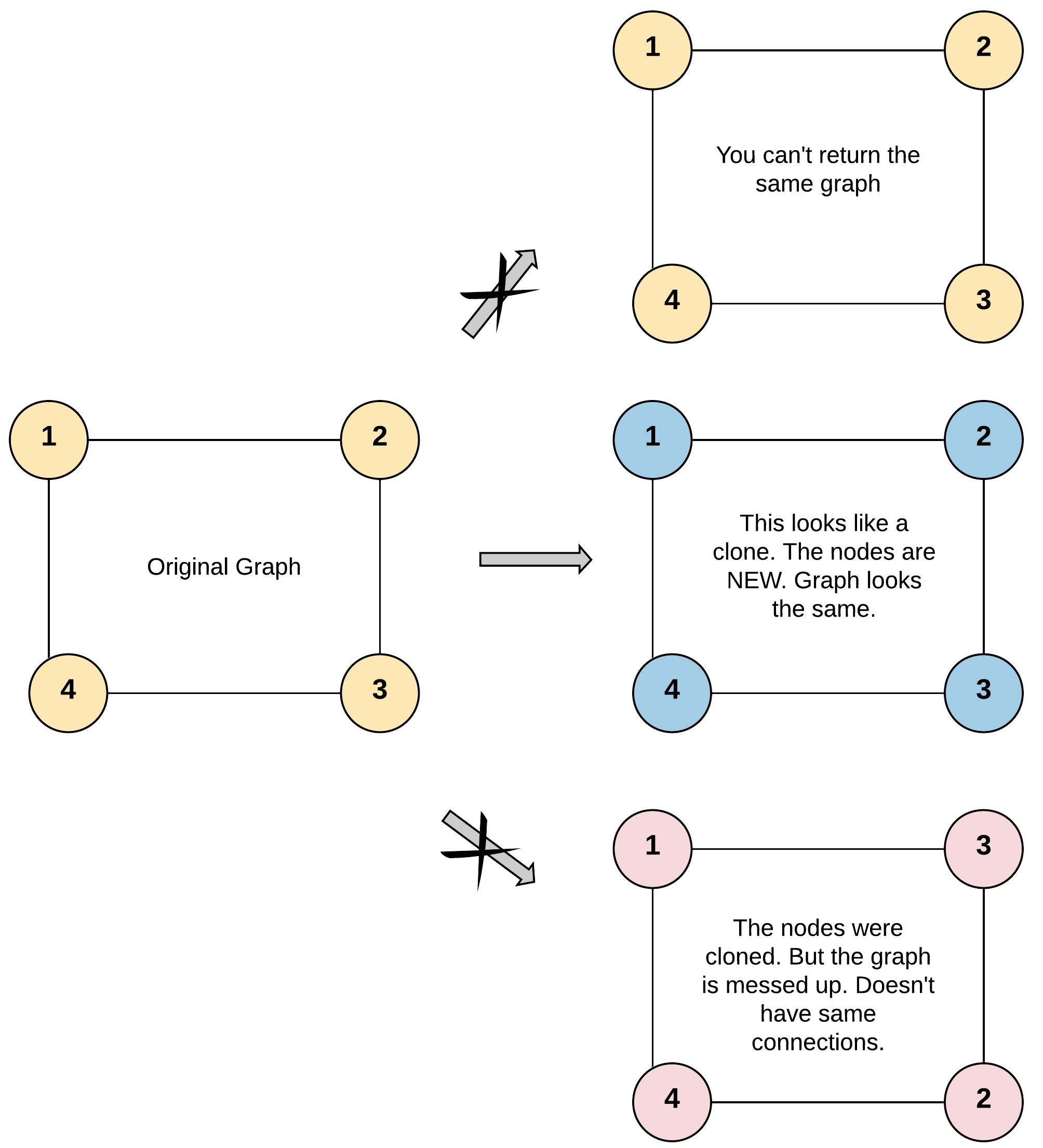Output: [[2,4],[1,3],[2,4],[1,3]]
Explanation: There are 4 nodes in the graph.
1st node (val = 1)’s neighbors are 2nd node (val = 2) and 4th node (val = 4).
2nd node (val = 2)’s neighbors are 1st node (val = 1) and 3rd node (val = 3).
3rd node (val = 3)’s neighbors are 2nd node (val = 2) and 4th node (val = 4).
4th node (val = 4)’s neighbors are 1st node (val = 1) and 3rd node (val = 3).

Constraints
The number of nodes in the graph is in the range [0, 100].
1 <= Node.val <= 100
Node.val is unique for each node.
There are no repeated edges and no self-loops in the graph.
The Graph is connected and all nodes can be visited starting from the given node.

dfs:

3刷：高频

Given two words (beginWord and endWord), and a dictionary’s word list, find the length of shortest transformation sequence from beginWord to endWord, such that:
Only one letter can be changed at a time.
Each transformed word must exist in the word list. Note that beginWord is not a transformed word.
Note:
Return 0 if there is no such transformation sequence.
All words have the same length.
All words contain only lowercase alphabetic characters.
You may assume no duplicates in the word list.
You may assume beginWord and endWord are non-empty and are not the same.

Example 1:
Input:
beginWord = “hit”,
endWord = “cog”,
wordList = [“hot”,”dot”,”dog”,”lot”,”log”,”cog”]
Output: 5
Explanation: As one shortest transformation is “hit” -> “hot” -> “dot” -> “dog” -> “cog”,
return its length 5.

Example 2:
Input:
beginWord = “hit”
endWord = “cog”
wordList = [“hot”,”dot”,”dog”,”lot”,”log”]
Output: 0
Explanation: The endWord “cog” is not in wordList, therefore no possible transformation.

### 785. Is Graph Bipartite? (Medium)

DFS:

BFS:

3刷：思路：用染色的方法，可以用 DFS, BFS 给所有 node 染上两种色中的一种。1.如未上色，上色，给相邻节点上相反色 2.如已上色，DFS: 要和当前要上的色相同 BFS：要和pop出来的颜色相反

### LinC 178. Graph Valid Tree (Medium)

Leetcode 261. Graph Valid Tree 带锁

### 675. Cut Off Trees for Golf Event (Hard)

You are asked to cut off trees in a forest for a golf event. The forest is represented as a non-negative 2D map, in this map:
0 represents the obstacle can’t be reached.
1 represents the ground can be walked through.
The place with number bigger than 1 represents a tree can be walked through, and this positive number represents the tree’s height.

You are asked to cut off all the trees in this forest in the order of tree’s height - always cut off the tree with lowest height first. And after cutting, the original place has the tree will become a grass (value 1).
You will start from the point (0, 0) and you should output the minimum steps you need to walk to cut off all the trees. If you can’t cut off all the trees, output -1 in that situation.
You are guaranteed that no two trees have the same height and there is at least one tree needs to be cut off.

### 310. Minimum Height Trees (Medium)

For an undirected graph with tree characteristics, we can choose any node as the root. The result graph is then a rooted tree. Among all possible rooted trees, those with minimum height are called minimum height trees (MHTs). Given such a graph, write a function to find all the MHTs and return a list of their root labels.
Format
The graph contains n nodes which are labeled from 0 to n - 1. You will be given the number n and a list of undirected edges (each edge is a pair of labels).
You can assume that no duplicate edges will appear in edges. Since all edges are undirected, [0, 1] is the same as [1, 0] and thus will not appear together in edges.

Note:
According to the definition of tree on Wikipedia: “a tree is an undirected graph in which any two vertices are connected by exactly one path. In other words, any connected graph without simple cycles is a tree.”
The height of a rooted tree is the number of edges on the longest downward path between the root and a leaf.

## Topological sorting 拓扑排序

### LinC 127. Topological Sorting (Medium)

Given an directed graph, a topological order of the graph nodes is defined as follow:

For each directed edge A -> B in graph, A must before B in the order list.
The first node in the order can be any node in the graph with no nodes direct to it.
Find any topological order for the given graph.
You can assume that there is at least one topological order in the graph.
Clarification

Example:
For graph as follow:The topological order can be:
[0, 1, 2, 3, 4, 5]
[0, 2, 3, 1, 5, 4]

### 207. Course Schedule (Medium)

There are a total of n courses you have to take, labeled from 0 to n-1.
Some courses may have prerequisites, for example to take course 0 you have to first take course 1, which is expressed as a pair: [0,1]
Given the total number of courses and a list of prerequisite pairs, is it possible for you to finish all courses?

Example 1:
Input: 2, [[1,0]]
Output: true
Explanation: There are a total of 2 courses to take.
To take course 1 you should have finished course 0. So it is possible.

Example 2:
Input: 2, [[1,0],[0,1]]
Output: false
Explanation: There are a total of 2 courses to take.
To take course 1 you should have finished course 0, and to take course 0 you should
also have finished course 1. So it is impossible.

Note:
The input prerequisites is a graph represented by a list of edges, not adjacency matrices. Read more about how a graph is represented.
You may assume that there are no duplicate edges in the input prerequisites.

3刷：面经：Cruise, 统计入度: …inDegree[e] += 1…

### 210. Course Schedule II (Medium)

There are a total of n courses you have to take, labeled from 0 to n-1.
Some courses may have prerequisites, for example to take course 0 you have to first take course 1, which is expressed as a pair: [0,1]
Given the total number of courses and a list of prerequisite pairs, return the ordering of courses you should take to finish all courses.
There may be multiple correct orders, you just need to return one of them. If it is impossible to finish all courses, return an empty array.

Example 1:
Input: 2, [[1,0]]
Output: [0,1]
Explanation: There are a total of 2 courses to take. To take course 1 you should have finished
course 0. So the correct course order is [0,1] .

Example 2:
Input: 4, [[1,0],[2,0],[3,1],[3,2]]
Output: [0,1,2,3] or [0,2,1,3]
Explanation: There are a total of 4 courses to take. To take course 3 you should have finished both
courses 1 and 2. Both courses 1 and 2 should be taken after you finished course 0.
So one correct course order is [0,1,2,3]. Another correct ordering is [0,2,1,3] .
Note:
The input prerequisites is a graph represented by a list of edges, not adjacency matrices. Read more about how a graph is represented.
You may assume that there are no duplicate edges in the input prerequisites.

2刷：面经：Cruise。时间复杂度：O(v + e) number of vertices and edges, 空间复杂度: O(v + e)

# DFS 深度优先搜索

## Binary Tree DFS 二叉树与树上的深度优先搜索

### 257. Binary Tree Paths (Easy)

Given a binary tree, return all root-to-leaf paths.
Note: A leaf is a node with no children.

Explanation: All root-to-leaf paths are: 1->2->5, 1->3

8刷：注意：1.剪枝，因为cur是一个reference，递归过程中cur会变长，当前层完成返回上一级函数调用时需要将当前层加上的多余的枝减去 2.ans.append()后不要return，不然无法剪枝

### 113. Path Sum II (Medium)

Given a binary tree and a sum, find all root-to-leaf paths where each path’s sum equals the given sum.
Note: A leaf is a node with no children.

9刷：高频，面经：Quora, 大疆。注意：ans.append()后面不要return，否则会丢失剪枝。TODO 用遍历再刷

### 437. Path Sum III (Medium)

Given the root of a binary tree and an integer targetSum, return the number of paths where the sum of the values along the path equals targetSum.

The path does not need to start or end at the root or a leaf, but it must go downwards (i.e., traveling only from parent nodes to child nodes).

Example 1: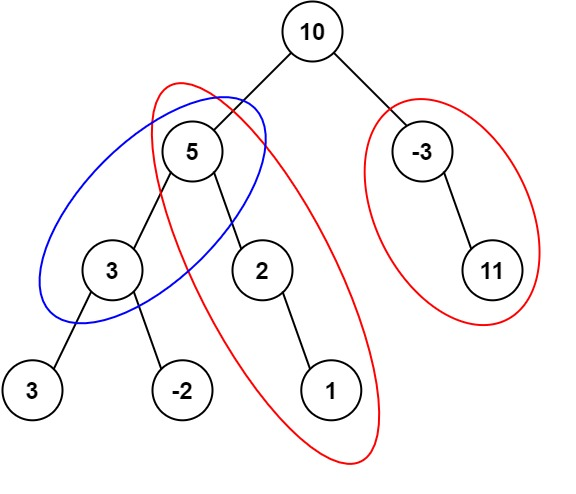Input: root = [10,5,-3,3,2,null,11,3,-2,null,1], targetSum = 8
Output: 3
Explanation: The paths that sum to 8 are shown.

Example 2:
Input: root = [5,4,8,11,null,13,4,7,2,null,null,5,1], targetSum = 22
Output: 3

Constraints:
The number of nodes in the tree is in the range [0, 1000].
-109 <= Node.val <= 109
-1000 <= targetSum <= 1000

O(n^2)

DFS + Memo：

7刷：面经：Quora。初始化memo为{0 : 1}的目的是当cur == sum的时候memo需要返回1因为这是一个符合条件的路径。 leetcode讨论区有详细的讨论

## Combination based DFS - 基于组合的深度优先搜索

### 78. Subsets (Medium)

Given a set of distinct integers, nums, return all possible subsets (the power set).
Note: The solution set must not contain duplicate subsets.

Example:
Input: nums = [1,2,3]
Output:
[
,
,
,
[1,2,3],
[1,3],
[2,3],
[1,2],
[]
]

7刷：高频。O(2^n)

### 39. Combination Sum (Medium)

Given a set of candidate numbers (candidates) (without duplicates) and a target number (target), find all unique combinations in candidates where the candidate numbers sums to target.
The same repeated number may be chosen from candidates unlimited number of times.
Note:
All numbers (including target) will be positive integers.
The solution set must not contain duplicate combinations.

7刷：高频, 面经, Quora, Amazon。需要排序的原因是为了避免结果里[2,3,2], [3,2,2]这类情况的发生，结果里只能取当前或比当前大的数。时间复杂度为O(n^k)，n是candidates的数量，k是target / min(candidates)或者用target来近似（假设min是1的话），这个上限比网上一些O(n!)的说法更低

### 40. Combination Sum II (Medium)

Given a collection of candidate numbers (candidates) and a target number (target), find all unique combinations in candidates where the candidate numbers sums to target.
Each number in candidates may only be used once in the combination.
Note:
All numbers (including target) will be positive integers.
The solution set must not contain duplicate combinations.

8刷：高频, 面经, amazon。o(2^n) 注意：…if i > idx and nums[i] == nums[i - 1]: continue…

### 216. combination sum iii (medium)

find all possible combinations of k numbers that add up to a number n, given that only numbers from 1 to 9 can be used and each combination should be a unique set of numbers.
note:
all numbers will be positive integers.
the solution set must not contain duplicate combinations.

6刷：面经

### 77. combinations (medium)

given two integers n and k, return all possible combinations of k numbers out of 1 … n.

example:
input: n = 4, k = 2
output:
[
[2,4],
[3,4],
[2,3],
[1,2],
[1,3],
[1,4],
]

4刷：面经：amazon

### 131. palindrome partitioning (medium)

given a string s, partition s such that every substring of the partition is a palindrome.
return all possible palindrome partitioning of s.

example:
input: “aab”
output:
[
[“aa”,”b”],
[“a”,”a”,”b”]
]

6刷：高频，o(n*(2^n))

### 93. restore ip addresses (medium)

given a string containing only digits, restore it by returning all possible valid ip address combinations.

example:
input: “25525511135”
output: [“255.255.11.135”, “255.255.111.35”]

11刷：高频。时间复杂度是一件有趣的事情，正常情况下分割字符串是o(2^n)复杂度，但是ip地址是有限的，因此这个题有个常数的时间复杂度上限

5刷

### 90. subsets ii (medium)

8刷：高频，无额外空间的解法需要if i > idx and …是因为要防止第一次就跳过重复的数字。在47题：permutations ii中因为全排列没有idx这个参数，需要用到用额外空间的写法去重

### 140. Word Break II (Hard)

Given a non-empty string s and a dictionary wordDict containing a list of non-empty words, add spaces in s to construct a sentence where each word is a valid dictionary word. Return all such possible sentences.

Note:

The same word in the dictionary may be reused multiple times in the segmentation.
You may assume the dictionary does not contain duplicate words.
Example 1:

Input:
s = “catsanddog”
wordDict = [“cat”, “cats”, “and”, “sand”, “dog”]
Output:
[
“cats and dog”,
“cat sand dog”
]
Example 2:

Input:
s = “pineapplepenapple”
wordDict = [“apple”, “pen”, “applepen”, “pine”, “pineapple”]
Output:
[
“pine apple pen apple”,
“pineapple pen apple”,
“pine applepen apple”
]
Explanation: Note that you are allowed to reuse a dictionary word.
Example 3:

Input:
s = “catsandog”
wordDict = [“cats”, “dog”, “sand”, “and”, “cat”]
Output:
[]

TLE:

AC: dfs + memo

6刷：面经：Amazon。九章。TODO 时间空间复杂度

### 698. Partition to K Equal Sum Subsets (Medium)

Given an integer array nums and an integer k, return true if it is possible to divide this array into k non-empty subsets whose sums are all equal.

Example 1:
Input: nums = [4,3,2,3,5,2,1], k = 4
Output: true
Explanation: It’s possible to divide it into 4 subsets (5), (1, 4), (2,3), (2,3) with equal sums.

Example 2:
Input: nums = [1,2,3,4], k = 3
Output: false

Constraints:
1 <= k <= nums.length <= 16
1 <= nums[i] <= 104
The frequency of each element is in the range [1, 4].

## Permutation based DFS - 基于排列的深度优先搜索

### 46. Permutations (Medium)

Given a collection of distinct integers, return all possible permutations.

Example:
Input: [1,2,3]
Output:
[
[1,2,3],
[1,3,2],
[2,1,3],
[2,3,1],
[3,1,2],
[3,2,1]
]

11刷：高频, used = [False] * len(nums); def dfs(). subset从idx开始往后扫，全排每一位都有可能放任意数字，因此要从第一位往后扫。这样就需要一个used的变量来记住哪一位已经被用过了

### 47. Permutations II (Medium)

Given a collection of numbers that might contain duplicates, return all possible unique permutations.

Example:
Input: [1,1,2]
Output:
[
[1,1,2],
[1,2,1],
[2,1,1]
]

13刷：高频，空间复杂度O(n)。时间复杂度：O(n*n!), 因为需要n步产生一个排列，总共有n！个可能的排列

### 22. Generate Parentheses (Medium)

Given n pairs of parentheses, write a function to generate all combinations of well-formed parentheses.

For example, given n = 3, a solution set is:
[
“((()))”,
“(()())”,
“(())()”,
“()(())”,
“()()()”
]

9刷：高频。时间复杂度分析涉及到nth catalan number的时间复杂度，超出面试范畴，如果是产生所有可能的括号时间复杂度是O(2^2n) != O(2^n)

### 51. N-Queens (Hard)

6刷：高频。检查下一行的某列的合法性：举个栗子就能看出来，检查列就看此列是否已在cur中（皇后已在此列），检查/方向的对角线是坐标相加，检查\方向的对角线是坐标相减，行递增无需检查。时间：O(n^3)， 空间：O(n)。因为c (count) 是row，v（value）是column, 正常 for c, v in enuerate() 就写成 for r, c in enumerate()

## Graph based DFS 基于图的深度优先搜索

### 17. Letter Combinations of a Phone Number (Medium)

Given a string containing digits from 2-9 inclusive, return all possible letter combinations that the number could represent.
A mapping of digit to letters (just like on the telephone buttons) is given below. Note that 1 does not map to any letters.Example:
Input: “23”
Output: [“ad”, “ae”, “af”, “bd”, “be”, “bf”, “cd”, “ce”, “cf”].

Note:
Although the above answer is in lexicographical order, your answer could be in any order you want.

8刷：高频

### 79. Word Search (Medium)

9刷：高频。这题的难点在于，递归函数内各方向的循环内不要做任何判断，判断需在循环外，这样才能走到len(word)的位置。而且由于r, c均可能出界，判断[idx] == [r][c]之前要先判断r，c是否出界

### 490. The Maze (Medium) 带锁

Lintcode 787. The Maze
There is a ball in a maze with empty spaces and walls. The ball can go through empty spaces by rolling up, down, left or right, but it won’t stop rolling until hitting a wall. When the ball stops, it could choose the next direction.

Given the ball’s start position, the destination and the maze, determine whether the ball could stop at the destination.

The maze is represented by a binary 2D array. 1 means the wall and 0 means the empty space. You may assume that the borders of the maze are all walls. The start and destination coordinates are represented by row and column indexes.

Example 1Example 2Note:
1.There is only one ball and one destination in the maze.
2.Both the ball and the destination exist on an empty space, and they will not be at the same position initially.
3.The given maze does not contain border (like the red rectangle in the example pictures), but you could assume the border of the maze are all walls.
4.The maze contains at least 2 empty spaces, and both the width and height of the maze won’t exceed 100.

7刷：面经：Amazon。TODO: BFS。确保不往回滚的关键是把滚到的终点标记为2，再往各可能方向滚好以后递归之前查看此点是否来过（为2)。for循环内要用nR, nC = r, c保存当前滚到的位置，才能四个方向都滚到

### 417. Pacific Atlantic Water Flow (Medium)

Given an m x n matrix of non-negative integers representing the height of each unit cell in a continent, the “Pacific ocean” touches the left and top edges of the matrix and the “Atlantic ocean” touches the right and bottom edges.
Water can only flow in four directions (up, down, left, or right) from a cell to another one with height equal or lower.
Find the list of grid coordinates where water can flow to both the Pacific and Atlantic ocean.

Note:
The order of returned grid coordinates does not matter.
Both m and n are less than 150.

dfs:

bfs:

4刷：面经：Cruise。从两个海接触的四条边上的点为起点往内陆灌，返回两个海都能灌到的点坐标

### 399. Evaluate Division (Medium)

Equations are given in the format A / B = k, where A and B are variables represented as strings, and k is a real number (floating point number). Given some queries, return the answers. If the answer does not exist, return -1.0.

Example:
Given a / b = 2.0, b / c = 3.0.
queries are: a / c = ?, b / a = ?, a / e = ?, a / a = ?, x / x = ? .
return [6.0, 0.5, -1.0, 1.0, -1.0 ].

The input is: vector<pair<string, string>> equations, vector& values, vector<pair<string, string>> queries , where equations.size() == values.size(), and the values are positive. This represents the equations. Return vector.

According to the example above:
equations = [ [“a”, “b”], [“b”, “c”] ],
values = [2.0, 3.0],
queries = [ [“a”, “c”], [“b”, “a”], [“a”, “e”], [“a”, “a”], [“x”, “x”] ].

The input is always valid. You may assume that evaluating the queries will result in no division by zero and there is no contradiction.

DFS:

BFS:

9刷：面经：头条。Currency Calculator一种题，先build graph，再用DFS, BFS寻最短路径的乘积

### 332. Reconstruct Itinerary (Medium)

Given a list of airline tickets represented by pairs of departure and arrival airports [from, to], reconstruct the itinerary in order. All of the tickets belong to a man who departs from JFK. Thus, the itinerary must begin with JFK.

Note:
If there are multiple valid itineraries, you should return the itinerary that has the smallest lexical order when read as a single string. For example, the itinerary [“JFK”, “LGA”] has a smaller lexical order than [“JFK”, “LGB”].
All airports are represented by three capital letters (IATA code).
You may assume all tickets form at least one valid itinerary.

Example 1:
Input: [[“MUC”, “LHR”], [“JFK”, “MUC”], [“SFO”, “SJC”], [“LHR”, “SFO”]]
Output: [“JFK”, “MUC”, “LHR”, “SFO”, “SJC”]

Example 2:
Input: [[“JFK”,”SFO”],[“JFK”,”ATL”],[“SFO”,”ATL”],[“ATL”,”JFK”],[“ATL”,”SFO”]]
Output: [“JFK”,”ATL”,”JFK”,”SFO”,”ATL”,”SFO”]
Explanation: Another possible reconstruction is [“JFK”,”SFO”,”ATL”,”JFK”,”ATL”,”SFO”].
But it is larger in lexical order.

2刷：高频

### 547. Number of Provinces (Medium)

There are n cities. Some of them are connected, while some are not. If city a is connected directly with city b, and city b is connected directly with city c, then city a is connected indirectly with city c.

A province is a group of directly or indirectly connected cities and no other cities outside of the group.

You are given an n x n matrix isConnected where isConnected[i][j] = 1 if the ith city and the jth city are directly connected, and isConnected[i][j] = 0 otherwise.

Return the total number of provinces.

Example 1:Input: isConnected = [[1,1,0],[1,1,0],[0,0,1]]
Output: 2

Example 2: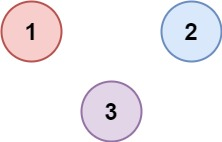Input: isConnected = [[1,0,0],[0,1,0],[0,0,1]]
Output: 3

Constraints:
1 <= n <= 200
n == isConnected.length
n == isConnected[i].length
isConnected[i][j] is 1 or 0.
isConnected[i][i] == 1
isConnected[i][j] == isConnected[j][i]

# 数据结构

## Array 数组

2刷，高频

### 56. Merge Intervals (Medium)

2刷，面经：Cruise。…cur = max(intervals[i], cur)

2刷：高频

### 48. Rotate Image (Medium)

2刷：高频：需要懂得顺时针转就是上下翻转以后对角线翻转，逆时针转就是左右翻转以后对角线翻转

4刷：高频

### 36. Valid Sudoku (Medium)

Determine if a 9x9 Sudoku board is valid. Only the filled cells need to be validated according to the following rules:
Each row must contain the digits 1-9 without repetition.
Each column must contain the digits 1-9 without repetition.
Each of the 9 3x3 sub-boxes of the grid must contain the digits 1-9 without repetition.
A partially filled sudoku which is valid.
The Sudoku board could be partially filled, where empty cells are filled with the character ‘.’.

Note:
A Sudoku board (partially filled) could be valid but is not necessarily solvable.
Only the filled cells need to be validated according to the mentioned rules.
The given board contain only digits 1-9 and the character ‘.’.
The given board size is always 9x9.

2刷：高频, 面经：维萨。*用来unpack数组，zip(*board)用来返回一个用每行同一列位置的元素拼成的新的tuple的iterator

4刷：高频

### 57. Insert Interval (Medium)

2刷：高频：写法1：用左右两个数组装不相交的区间，处理相交的区间，将左右和新区间拼接。 写法2：先把结束小于新开始的加到ans里；然后过开始小于等于新结束的，合并区间，加到ans中；最后把剩下的加到ans

### 189. Rotate Array (Easy)

Given an array, rotate the array to the right by k steps, where k is non-negative.

Note:
Try to come up as many solutions as you can, there are at least 3 different ways to solve this problem.
Could you do it in-place with O(1) extra space?

O(n) space:

O(1) space:

### 296. Best Meeting Point (Hard) 带锁

LinC 912. Best Meeting Point
A group of two or more people wants to meet and minimize the total travel distance. You are given a 2D grid of values 0 or 1, where each 1 marks the home of someone in the group. The distance is calculated using Manhattan Distance, where distance(p1, p2) = |p2.x - p1.x| + |p2.y - p1.y|.

Example 1:
Input:
[[1,0,0,0,1],[0,0,0,0,0],[0,0,1,0,0]]
Output:
6
Explanation:
The point `(0,2)` is an ideal meeting point, as the total travel distance of `2 + 2 + 2 = 6` is minimal. So return `6`.

Example 2:
Input:
[[1,1,0,0,1],[1,0,1,0,0],[0,0,1,0,1]]
Output:
14

## Sliding Window 滑动窗口

### 438. Find All Anagrams in a String (Medium)

Given a string s and a non-empty string p, find all the start indices of p’s anagrams in s.
Strings consists of lowercase English letters only and the length of both strings s and p will not be larger than 20,100.
The order of output does not matter.

Example 1:
Input:
s: “cbaebabacd” p: “abc”
Output:
[0, 6]
Explanation:
The substring with start index = 0 is “cba”, which is an anagram of “abc”.
The substring with start index = 6 is “bac”, which is an anagram of “abc”.

Example 2:
Input:
s: “abab” p: “ab”
Output:
[0, 1, 2]
Explanation:
The substring with start index = 0 is “ab”, which is an anagram of “ab”.
The substring with start index = 1 is “ba”, which is an anagram of “ab”.
The substring with start index = 2 is “ab”, which is an anagram of “ab”.

2刷：面经：Amazon

### 3. Longest Substring Without Repeating Characters (Medium)

Given a string, find the length of the longest substring without repeating characters.

Example 1:
Input: “abcabcbb”
Output: 3
Explanation: The answer is “abc”, with the length of 3.

Example 2:
Input: “bbbbb”
Output: 1
Explanation: The answer is “b”, with the length of 1.

Example 3:
Input: “pwwkew”
Output: 3
Explanation: The answer is “wke”, with the length of 3.
Note that the answer must be a substring, “pwke” is a subsequence and not a substring.

6刷：面经，Amazon

### 76. Minimum Window Substring (Hard)

Given a string S and a string T, find the minimum window in S which will contain all the characters in T in complexity O(n).

Example:
Input: S = “ADOBECODEBANC”, T = “ABC”
Output: “BANC”

Note:
If there is no such window in S that covers all characters in T, return the empty string “”.
If there is such window, you are guaranteed that there will always be only one unique minimum window in S.

3刷：面经, Amazon

### 209. Minimum Size Subarray Sum (Medium)

Given an array of positive integers nums and a positive integer target, return the minimal length of a contiguous subarray [numsl, numsl+1, …, numsr-1, numsr] of which the sum is greater than or equal to target. If there is no such subarray, return 0 instead.

Example 1:
Input: target = 7, nums = [2,3,1,2,4,3]
Output: 2
Explanation: The subarray [4,3] has the minimal length under the problem constraint.

Example 2:
Input: target = 4, nums = [1,4,4]
Output: 1

Example 3:
Input: target = 11, nums = [1,1,1,1,1,1,1,1]
Output: 0

Constraints:
1 <= target <= 109
1 <= nums.length <= 105
1 <= nums[i] <= 105

Follow up: If you have figured out the O(n) solution, try coding another solution of which the time complexity is O(n log(n)).

### 1151 Minimum Swaps to Group All 1’s Together (Medium) 带锁

geeks for geeks article
Given an array of 0’s and 1’s, we need to write a program to find the minimum number of swaps required to group all 1’s present in the array together.

Examples:
Input : arr[] = {1, 0, 1, 0, 1}
Output : 1
Explanation: Only 1 swap is required to
group all 1’s together. Swapping index 1
and 4 will give arr[] = {1, 1, 1, 0, 0}

Input : arr[] = {1, 0, 1, 0, 1, 1}
Output : 1

### 1004. Max Consecutive Ones III medium

Given an array A of 0s and 1s, we may change up to K values from 0 to 1.
Return the length of the longest (contiguous) subarray that contains only 1s.

Example 1:
Input: A = [1,1,1,0,0,0,1,1,1,1,0], K = 2
Output: 6
Explanation:
[1,1,1,0,0,1,1,1,1,1,1]
Bolded numbers were flipped from 0 to 1. The longest subarray is underlined.

Example 2:
Input: A = [0,0,1,1,0,0,1,1,1,0,1,1,0,0,0,1,1,1,1], K = 3
Output: 10
Explanation:
[0,0,1,1,1,1,1,1,1,1,1,1,0,0,0,1,1,1,1]
Bolded numbers were flipped from 0 to 1. The longest subarray is underlined.

Note:
1 <= A.length <= 20000
0 <= K <= A.length
A[i] is 0 or 1

### [83. Remove Duplicates from Sorted List (Easy)](83. Remove Duplicates from Sorted List)

Given a sorted linked list, delete all duplicates such that each element appear only once.

Example 1:
Input: 1->1->2
Output: 1->2

Example 2:
Input: 1->1->2->3->3
Output: 1->2->3

### 82. Remove Duplicates from Sorted List II (Medium)

Given a sorted linked list, delete all nodes that have duplicate numbers, leaving only distinct numbers from the original list.

Example 1:
Input: 1->2->3->3->4->4->5
Output: 1->2->5

Example 2:
Input: 1->1->1->2->3
Output: 2->3

### 21. Merge Two Sorted Lists (Easy)

Merge two sorted linked lists and return it as a new list. The new list should be made by splicing together the nodes of the first two lists.

Example:
Input: 1->2->4, 1->3->4
Output: 1->1->2->3->4->4

### 19. Remove Nth Node From End of List (Medium)

Given a linked list, remove the n-th node from the end of list and return its head.

Example:
Given linked list: 1->2->3->4->5, and n = 2.
After removing the second node from the end, the linked list becomes 1->2->3->5.

Note:
Given n will always be valid.

Could you do this in one pass?

### 2. Add Two Numbers (Medium)

2刷：总结：写l2n和n2l两个函数

### 445. Add Two Numbers II (Medium)

You are given two non-empty linked lists representing two non-negative integers. The most significant digit comes first and each of their nodes contains a single digit. Add the two numbers and return the sum as a linked list.

You may assume the two numbers do not contain any leading zero, except the number 0 itself.

Example 1: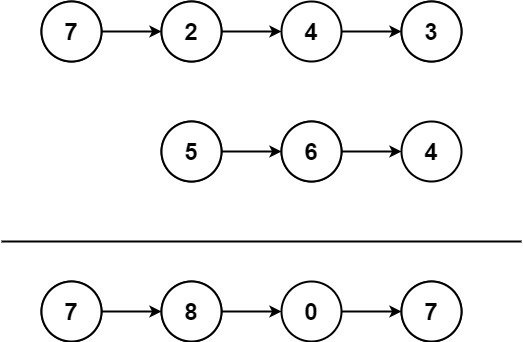Input: l1 = [7,2,4,3], l2 = [5,6,4]
Output: [7,8,0,7]

Example 2:
Input: l1 = [2,4,3], l2 = [5,6,4]
Output: [8,0,7]

Example 3:
Input: l1 = , l2 = 
Output: 

Constraints:
The number of nodes in each linked list is in the range [1, 100].
0 <= Node.val <= 9
It is guaranteed that the list represents a number that does not have leading zeros.

Follow up: Could you solve it without reversing the input lists?

stack:

### 86. Partition List (Medium)

Given a linked list and a value x, partition it such that all nodes less than x come before nodes greater than or equal to x.
You should preserve the original relative order of the nodes in each of the two partitions.

Example:
Input: head = 1->4->3->2->5->2, x = 3
Output: 1->2->2->4->3->5

### 141. Linked List Cycle (Easy)

Given a linked list, determine if it has a cycle in it.
To represent a cycle in the given linked list, we use an integer pos which represents the position (0-indexed) in the linked list where tail connects to. If pos is -1, then there is no cycle in the linked list.

Example 1:
Input: head = [3,2,0,-4], pos = 1
Output: true
Explanation: There is a cycle in the linked list, where tail connects to the second node.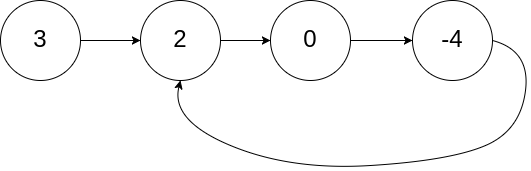Example 2:
Input: head = [1,2], pos = 0
Output: true
Explanation: There is a cycle in the linked list, where tail connects to the first node.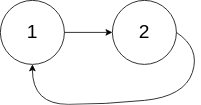Example 3:
Input: head = , pos = -1
Output: false
Explanation: There is no cycle in the linked list.Can you solve it without using extra space?

### 142. Linked List Cycle II (Medium)

Given a linked list, return the node where the cycle begins. If there is no cycle, return null.
To represent a cycle in the given linked list, we use an integer pos which represents the position (0-indexed) in the linked list where tail connects to. If pos is -1, then there is no cycle in the linked list.
Note: Do not modify the linked list.

Example 1:
Input: head = [3,2,0,-4], pos = 1
Output: tail connects to node index 1
Explanation: There is a cycle in the linked list, where tail connects to the second node.Example 2:
Input: head = [1,2], pos = 0
Output: tail connects to node index 0
Explanation: There is a cycle in the linked list, where tail connects to the first node.Example 3:
Input: head = , pos = -1
Output: no cycle
Explanation: There is no cycle in the linked list.### 206. Reverse Linked List (Easy)

Example:
Input: 1->2->3->4->5->NULL
Output: 5->4->3->2->1->NULL

A linked list can be reversed either iteratively or recursively. Could you implement both?

### 92. Reverse Linked List II (Medium)

Reverse a linked list from position m to n. Do it in one-pass.
Note: 1 ≤ m ≤ n ≤ length of list.

Example:
Input: 1->2->3->4->5->NULL, m = 2, n = 4
Output: 1->4->3->2->5->NULL

3刷：高频

### 160. Intersection of Two Linked Lists (Easy)

Write a program to find the node at which the intersection of two singly linked lists begins.
For example, the following two linked lists:

begin to intersect at node c1.

Notes:
If the two linked lists have no intersection at all, return null.
The linked lists must retain their original structure after the function returns.
You may assume there are no cycles anywhere in the entire linked structure.
Your code should preferably run in O(n) time and use only O(1) memory.

2刷：思路：统计两条链走到头的长度，lenA 和 lenB, 然后让长的那条先走两者的差值，然后一起走，返回相遇的那点

### 24. Swap Nodes in Pairs (Medium)

You may not modify the values in the list’s nodes, only nodes itself may be changed.

Example:
Given 1->2->3->4, you should return the list as 2->1->4->3.

### 61. Rotate List (Medium)

Given a linked list, rotate the list to the right by k places, where k is non-negative.

Example 1:
Input: 1->2->3->4->5->NULL, k = 2
Output: 4->5->1->2->3->NULL
Explanation:
rotate 1 steps to the right: 5->1->2->3->4->NULL
rotate 2 steps to the right: 4->5->1->2->3->NULL

Example 2:
Input: 0->1->2->NULL, k = 4
Output: 2->0->1->NULL
Explanation:
rotate 1 steps to the right: 2->0->1->NULL
rotate 2 steps to the right: 1->2->0->NULL
rotate 3 steps to the right: 0->1->2->NULL
rotate 4 steps to the right: 2->0->1->NULL

### 146. LRU Cache (Medium)

Design and implement a data structure for Least Recently Used (LRU) cache. It should support the following operations: get and put.

get(key) - Get the value (will always be positive) of the key if the key exists in the cache, otherwise return -1.
put(key, value) - Set or insert the value if the key is not already present. When the cache reached its capacity, it should invalidate the least recently used item before inserting a new item.
The cache is initialized with a positive capacity.

Could you do both operations in O(1) time complexity?

7刷：高频, 注意put的时候要先把旧的重复key对从双链表中删除。或者使用OrderedDict这个数据结构，支持头部删除(mapping.popitem(last = False))和move_to_end(key)

### 234. Palindrome Linked List (Easy)

3刷：高频, 慢指针边走边反转，当链表是奇数个时需要s跳过中间节点再s,pre_h同时前进：…if f: s = s.next…

## Stack 栈

### 20. Valid Parentheses (Easy)

2刷：高频：注意在出栈前检验栈是否为空，走完以后检查栈是否为空

### 155. Min Stack (Easy)

2刷：高频：一个stack就用tuple，两个stack就相当于第二个stack来维持curMin

### 224. Basic Calculator (Hard)

Implement a basic calculator to evaluate a simple expression string.
The expression string may contain open ( and closing parentheses ), the plus + or minus sign -, non-negative integers and empty spaces .

Example 1:
Input: “1 + 1”
Output: 2
Example 2:

Input: “ 2-1 + 2 “
Output: 3
Example 3:

Input: “(1+(4+5+2)-3)+(6+8)”
Output: 23
Note:
You may assume that the given expression is always valid.
Do not use the eval built-in library function.

### 227. Basic Calculator II (Medium)

Implement a basic calculator to evaluate a simple expression string.
The expression string contains only non-negative integers, +, -, * , / operators and empty spaces . The integer division should truncate toward zero.

Example 1:
Input: “3+2*2”
Output: 7
Example 2:

Input: “ 3/2 “
Output: 1
Example 3:

Input: “ 3+5 / 2 “
Output: 5

Note:
You may assume that the given expression is always valid.
Do not use the eval built-in library function.

O(n) 空间:

O(1）空间:

6刷: 面经：Cruise。难点:
1：preOp = …; …in s + ‘+’
2. 给s加一个’+’尾巴处理最后一个运算符
3. -3 // 2 == -2 因为python是floor division返回less or equal to the closest integer比-1.5 less的integer是-2，所以要用int(../..)这样来除才能得到整数位的
4：O(n）空间的写法是要有一个preV的变量记录前一位的结果，如果前一个运算符是加减，则将preV加入res，最后返回res + preV

stack:

3刷：高频